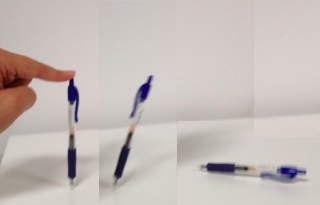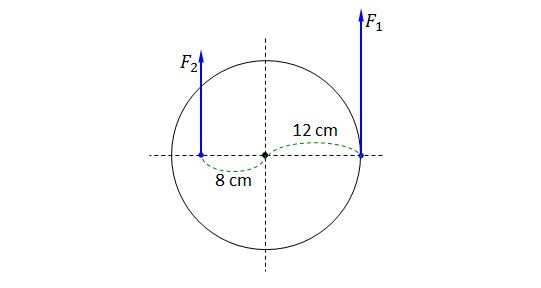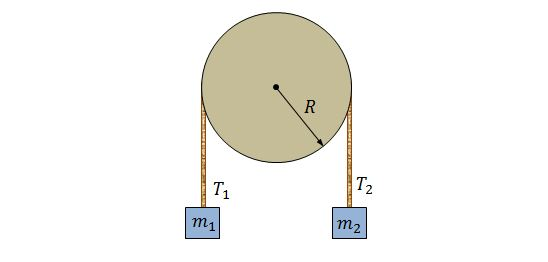Classical Mechanics

# Rotational Form of Newton's Second LawI assume that you work through these problems using some sort of writing utensil. Take your pen, balance it on its tip, and let go. It falls over. How fast in m/s is the other end of the pen going when it hits the table, assuming the tip doesn't slip? Take the pen to be a uniform one dimensional rod of length 15 cm.

If an angular acceleration of $$28.0\text{ rad/s}^2$$ is generated by a $$44.0\text{ N}\cdot\text{m}$$ torque on a wheel, then what is the wheel's approximate rotational inertia?

Consider a diver who is launching from a diving board. When he launches from the board, his angular speed about his center of mass is changing from $$0$$ to $$5.35\text{ rad/s}$$ in $$190\text{ ms}.$$ If the rotational inertia about his center of mass is $$12.0\text{ kg}\cdot\text{m}^2,$$ what is the magnitude of average external torque on him from the board during the launch?A cylinder is pinned at its center and two forces $$F_1=110\text{ N}$$ and $$F_2=70\text{ N}$$ are acting on it, as shown above. If the mass of the cylinder is $$100\text{ kg},$$ what is the rotational acceleration produced by the two forces?Two blocks of masses $$m_1=430\text{ g}$$ and $$m_2=500\text{ g}$$ are hanging on a pulley, as shown in the above figure. The pulley is fixed on a horizontal axle with negligible friction, and its radius is $$R=5.00\text{ cm}.$$ If they are released from rest, block $$m_2$$ falls $$48.0\text{ cm}$$ in $$4.00\text{ s}.$$ If there is no slippage between the cord and the pulley, what is the rotational inertia of the pulley?

The gravitational acceleration is $$g=9.8\text{ m/s}^2.$$

×

Problem Loading...

Note Loading...

Set Loading...